# PivotGridFieldSortBySummaryInfo.SummaryType Property

Gets or sets the summary function type used to calculate the summary values which define the order in which the current column field’s (row field’s) values are arranged.

Namespace: DevExpress.XtraPivotGrid

Assembly: DevExpress.PivotGrid.v22.2.Core.dll

NuGet Packages: DevExpress.PivotGrid.Core, DevExpress.Win.Dashboard.Design

## Declaration

``````[DefaultValue(PivotSummaryType.Sum)]
public PivotSummaryType SummaryType { get; set; }``````

## Property Value

Type Default Description
PivotSummaryType Sum

A PivotSummaryType value that specifies the summary function type.

Available values:

Show 13 items
Name Description
Count

The number of values (excluding Null and DBNull values).

Sum

The sum of the values.Min

The smallest value.

Max

The largest value.

Average

The average of the values.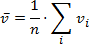StdDev

An estimate of the standard deviation of a population, where the sample is a subset of the entire population.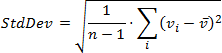StdDevp

The standard deviation of a population, where the population is all of the data to be summarized.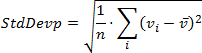Var

An estimate of the variance of a population, where the sample is a subset of the entire population.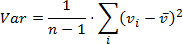Varp

The variance of a population, where the population is all of the data to be summarized.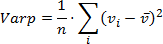Custom

Specifies whether calculations should be performed manually, by handling the CustomSummary event.

Not supported in OLAP and server mode.

CountDistinct

The number of distinct values. This function works only in the Optimized mode.

Median

The median of the values. A median is the number separating the higher half of a value range from the lower half. This function works only in the Optimized mode.

Mode

The mode of the values. A mode is the value that is repeated more often than any other. This function works only in the Optimized mode.

## Remarks

The PivotGridFieldSortBySummaryInfo.FieldName and SummaryType properties should be used together to specify the field and summary function type. This summary function will calculated against this field and the summary values will define the order in which the values of the current column field or row field will be arranged

The PivotGridFieldSortBySummaryInfo.FieldName and SummaryType properties are in effect if the PivotGridFieldSortBySummaryInfo.Field property is set to null.

The following code snippet (auto-collected from DevExpress Examples) contains a reference to the SummaryType property.

Note

The algorithm used to collect these code examples remains a work in progress. Accordingly, the links and snippets below may produce inaccurate results. If you encounter an issue with code examples below, please use the feedback form on this page to report the issue.